Anúncio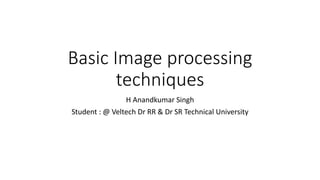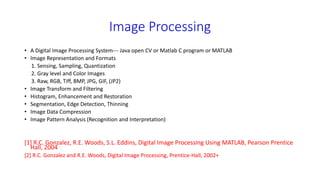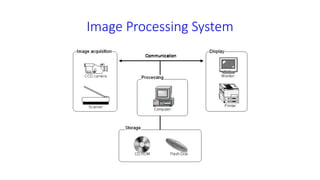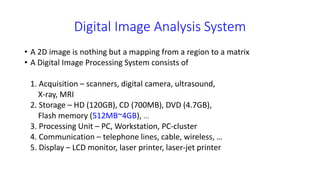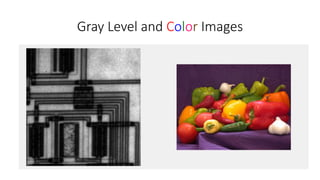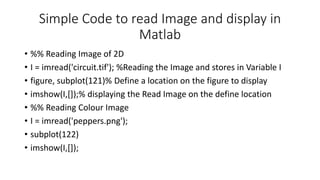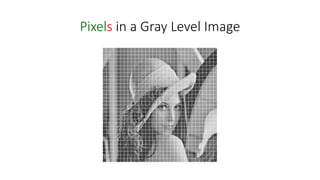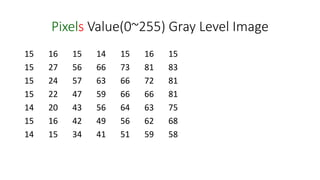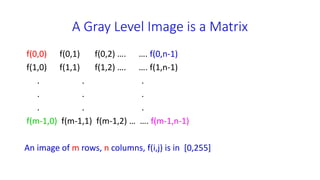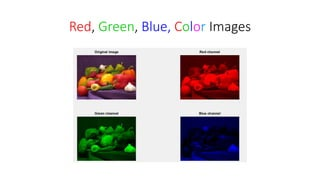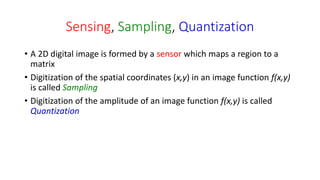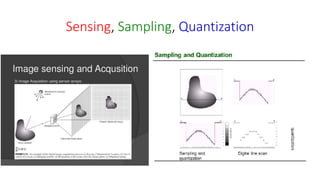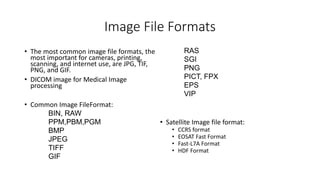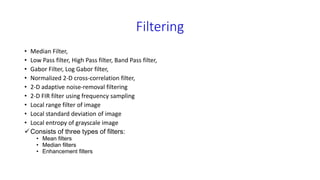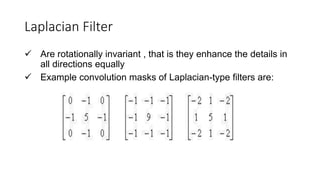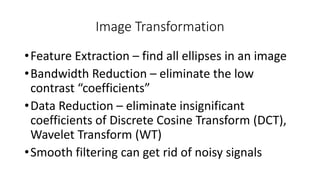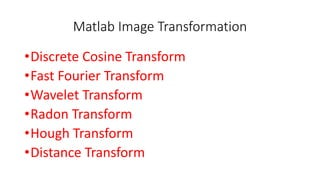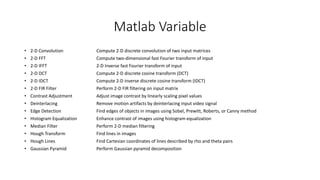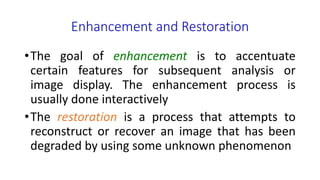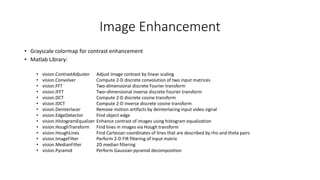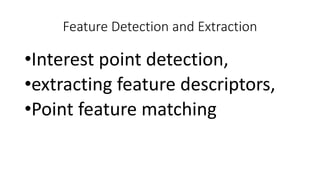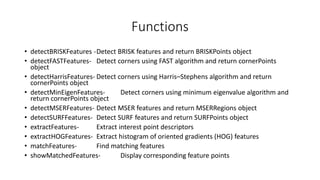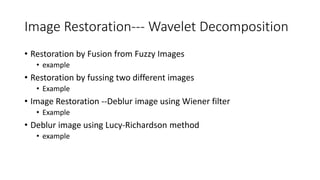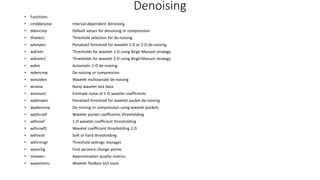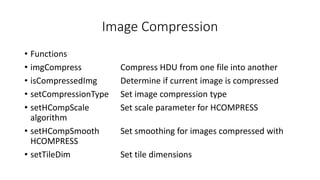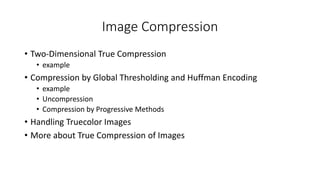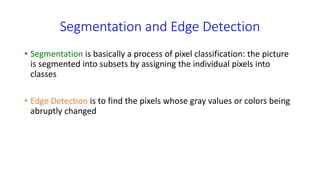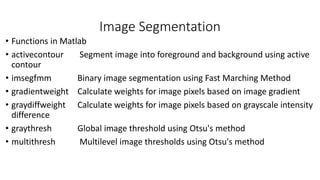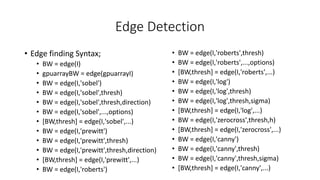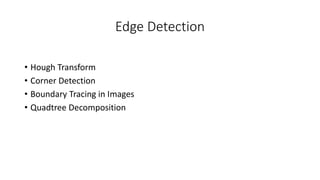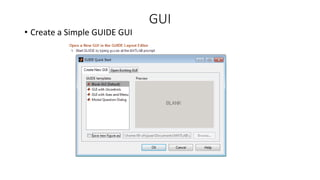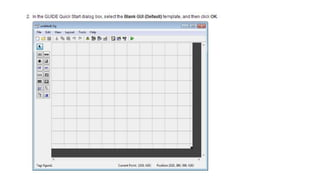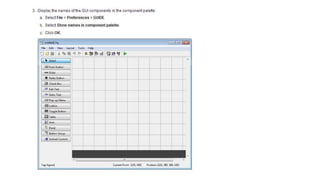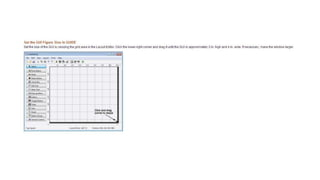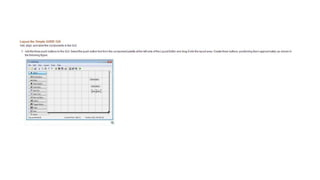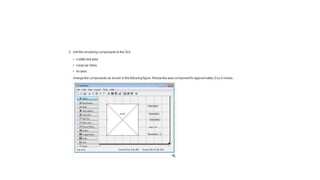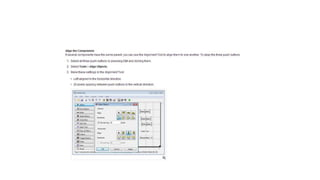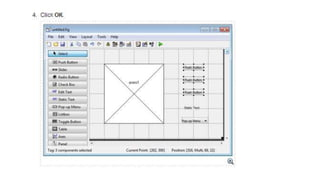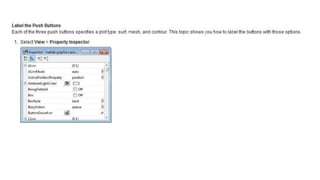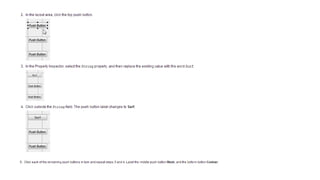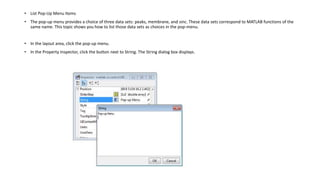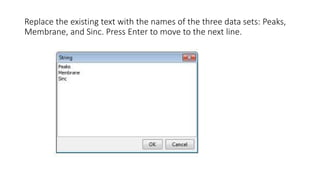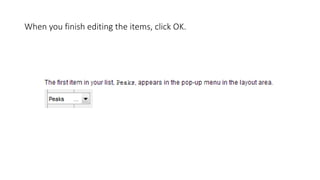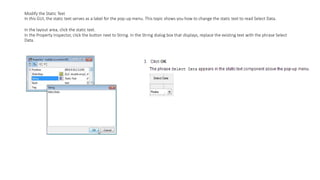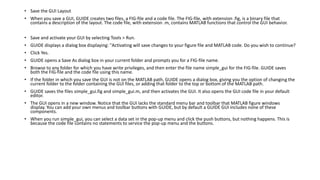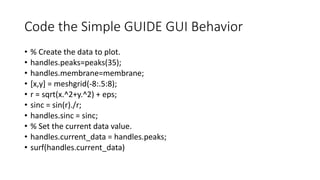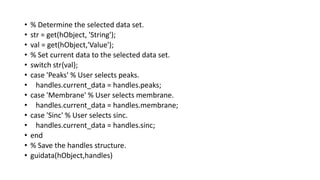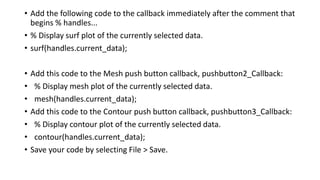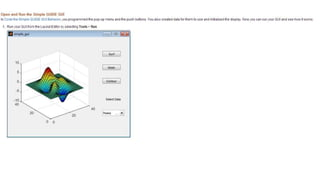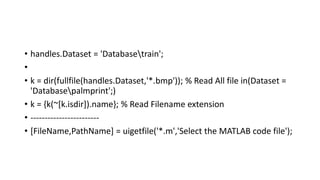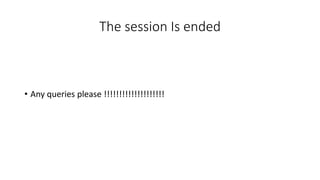1 de 51
Anúncio

### Basic image processing techniques

1. Basic Image processing techniques H Anandkumar Singh Student : @ Veltech Dr RR & Dr SR Technical University
2. Image Processing • A Digital Image Processing System--- Java open CV or Matlab C program or MATLAB • Image Representation and Formats 1. Sensing, Sampling, Quantization 2. Gray level and Color Images 3. Raw, RGB, Tiff, BMP, JPG, GIF, (JP2) • Image Transform and Filtering • Histogram, Enhancement and Restoration • Segmentation, Edge Detection, Thinning • Image Data Compression • Image Pattern Analysis (Recognition and Interpretation)  R.C. Gonzalez, R.E. Woods, S.L. Eddins, Digital Image Processing Using MATLAB, Pearson Prentice Hall, 2004  R.C. Gonzalez and R.E. Woods, Digital Image Processing, Prentice-Hall, 2002+
3. Image Processing System
4. Digital Image Analysis System • A 2D image is nothing but a mapping from a region to a matrix • A Digital Image Processing System consists of 1. Acquisition – scanners, digital camera, ultrasound, X-ray, MRI 2. Storage – HD (120GB), CD (700MB), DVD (4.7GB), Flash memory (512MB~4GB), … 3. Processing Unit – PC, Workstation, PC-cluster 4. Communication – telephone lines, cable, wireless, … 5. Display – LCD monitor, laser printer, laser-jet printer
5. Gray Level and Color Images
6. Simple Code to read Image and display in Matlab • %% Reading Image of 2D • I = imread('circuit.tif'); %Reading the Image and stores in Variable I • figure, subplot(121)% Define a location on the figure to display • imshow(I,[]);% displaying the Read Image on the define location • %% Reading Colour Image • I = imread('peppers.png'); • subplot(122) • imshow(I,[]);
7. Pixels in a Gray Level Image
8. Pixels Value(0~255) Gray Level Image 15 16 15 14 15 16 15 15 27 56 66 73 81 83 15 24 57 63 66 72 81 15 22 47 59 66 66 81 14 20 43 56 64 63 75 15 16 42 49 56 62 68 14 15 34 41 51 59 58
9. A Gray Level Image is a Matrix f(0,0) f(0,1) f(0,2) …. …. f(0,n-1) f(1,0) f(1,1) f(1,2) …. …. f(1,n-1) . . . . . . . . . f(m-1,0) f(m-1,1) f(m-1,2) … …. f(m-1,n-1) An image of m rows, n columns, f(i,j) is in [0,255]
10. Red, Green, Blue, Color Images
11. Sensing, Sampling, Quantization • A 2D digital image is formed by a sensor which maps a region to a matrix • Digitization of the spatial coordinates (x,y) in an image function f(x,y) is called Sampling • Digitization of the amplitude of an image function f(x,y) is called Quantization
12. Sensing, Sampling, Quantization
13. Image File Formats • The most common image file formats, the most important for cameras, printing, scanning, and internet use, are JPG, TIF, PNG, and GIF. • DICOM image for Medical Image processing • Common Image FileFormat: BIN, RAW PPM,PBM,PGM BMP JPEG TIFF GIF RAS SGI PNG PICT, FPX EPS VIP • Satellite Image file format: • CCRS format • EOSAT Fast Format • Fast-L7A Format • HDF Format
14. Filtering • Median Filter, • Low Pass filter, High Pass filter, Band Pass filter, • Gabor Filter, Log Gabor filter, • Normalized 2-D cross-correlation filter, • 2-D adaptive noise-removal filtering • 2-D FIR filter using frequency sampling • Local range filter of image • Local standard deviation of image • Local entropy of grayscale image Consists of three types of filters: • Mean filters • Median filters • Enhancement filters
15. Laplacian Filter  Are rotationally invariant , that is they enhance the details in all directions equally  Example convolution masks of Laplacian-type filters are:
16. Image Transformation •Feature Extraction – find all ellipses in an image •Bandwidth Reduction – eliminate the low contrast “coefficients” •Data Reduction – eliminate insignificant coefficients of Discrete Cosine Transform (DCT), Wavelet Transform (WT) •Smooth filtering can get rid of noisy signals
17. Matlab Image Transformation •Discrete Cosine Transform •Fast Fourier Transform •Wavelet Transform •Radon Transform •Hough Transform •Distance Transform
18. Matlab Variable • 2-D Convolution Compute 2-D discrete convolution of two input matrices • 2-D FFT Compute two-dimensional fast Fourier transform of input • 2-D IFFT 2-D Inverse fast Fourier transform of input • 2-D DCT Compute 2-D discrete cosine transform (DCT) • 2-D IDCT Compute 2-D inverse discrete cosine transform (IDCT) • 2-D FIR Filter Perform 2-D FIR filtering on input matrix • Contrast Adjustment Adjust image contrast by linearly scaling pixel values • Deinterlacing Remove motion artifacts by deinterlacing input video signal • Edge Detection Find edges of objects in images using Sobel, Prewitt, Roberts, or Canny method • Histogram Equalization Enhance contrast of images using histogram equalization • Median Filter Perform 2-D median filtering • Hough Transform Find lines in images • Hough Lines Find Cartesian coordinates of lines described by rho and theta pairs • Gaussian Pyramid Perform Gaussian pyramid decomposition
19. Enhancement and Restoration •The goal of enhancement is to accentuate certain features for subsequent analysis or image display. The enhancement process is usually done interactively •The restoration is a process that attempts to reconstruct or recover an image that has been degraded by using some unknown phenomenon
20. Image Enhancement • Grayscale colormap for contrast enhancement • Matlab Library: • vision.ContrastAdjuster Adjust image contrast by linear scaling • vision.Convolver Compute 2-D discrete convolution of two input matrices • vision.FFT Two-dimensional discrete Fourier transform • vision.IFFT Two–dimensional inverse discrete Fourier transform • vision.DCT Compute 2-D discrete cosine transform • vision.IDCT Compute 2-D inverse discrete cosine transform • vision.Deinterlacer Remove motion artifacts by deinterlacing input video signal • vision.EdgeDetector Find object edge • vision.HistogramEqualizer Enhance contrast of images using histogram equalization • vision.HoughTransform Find lines in images via Hough transform • vision.HoughLines Find Cartesian coordinates of lines that are described by rho and theta pairs • vision.ImageFilter Perform 2-D FIR filtering of input matrix • vision.MedianFilter 2D median filtering • vision.Pyramid Perform Gaussian pyramid decomposition
21. Feature Detection and Extraction •Interest point detection, •extracting feature descriptors, •Point feature matching
22. Functions • detectBRISKFeatures -Detect BRISK features and return BRISKPoints object • detectFASTFeatures- Detect corners using FAST algorithm and return cornerPoints object • detectHarrisFeatures- Detect corners using Harris–Stephens algorithm and return cornerPoints object • detectMinEigenFeatures- Detect corners using minimum eigenvalue algorithm and return cornerPoints object • detectMSERFeatures- Detect MSER features and return MSERRegions object • detectSURFFeatures- Detect SURF features and return SURFPoints object • extractFeatures- Extract interest point descriptors • extractHOGFeatures- Extract histogram of oriented gradients (HOG) features • matchFeatures- Find matching features • showMatchedFeatures- Display corresponding feature points
23. Image Restoration--- Wavelet Decomposition • Restoration by Fusion from Fuzzy Images • example • Restoration by fussing two different images • Example • Image Restoration --Deblur image using Wiener filter • Example • Deblur image using Lucy-Richardson method • example
24. Denoising • Functions • cmddenoise Interval-dependent denoising • ddencmp Default values for denoising or compression • thselect Threshold selection for de-noising • wbmpen Penalized threshold for wavelet 1-D or 2-D de-noising • wdcbm Thresholds for wavelet 1-D using Birgé-Massart strategy • wdcbm2 Thresholds for wavelet 2-D using Birgé-Massart strategy • wden Automatic 1-D de-noising • wdencmp De-noising or compression • wmulden Wavelet multivariate de-noising • wnoise Noisy wavelet test data • wnoisest Estimate noise of 1-D wavelet coefficients • wpbmpen Penalized threshold for wavelet packet de-noising • wpdencmp De-noising or compression using wavelet packets • wpthcoef Wavelet packet coefficients thresholding • wthcoef 1-D wavelet coefficient thresholding • wthcoef2 Wavelet coefficient thresholding 2-D • wthresh Soft or hard thresholding • wthrmngr Threshold settings manager • wvarchg Find variance change points • measerr Approximation quality metrics • wavemenu Wavelet Toolbox GUI tools
25. Image Compression • Functions • imgCompress Compress HDU from one file into another • isCompressedImg Determine if current image is compressed • setCompressionType Set image compression type • setHCompScale Set scale parameter for HCOMPRESS algorithm • setHCompSmooth Set smoothing for images compressed with HCOMPRESS • setTileDim Set tile dimensions
26. Image Compression • Two-Dimensional True Compression • example • Compression by Global Thresholding and Huffman Encoding • example • Uncompression • Compression by Progressive Methods • Handling Truecolor Images • More about True Compression of Images
27. Segmentation and Edge Detection • Segmentation is basically a process of pixel classification: the picture is segmented into subsets by assigning the individual pixels into classes • Edge Detection is to find the pixels whose gray values or colors being abruptly changed
28. Image Segmentation • Functions in Matlab • activecontour Segment image into foreground and background using active contour • imsegfmm Binary image segmentation using Fast Marching Method • gradientweight Calculate weights for image pixels based on image gradient • graydiffweight Calculate weights for image pixels based on grayscale intensity difference • graythresh Global image threshold using Otsu's method • multithresh Multilevel image thresholds using Otsu's method
29. Edge Detection • Edge finding Syntax; • BW = edge(I) • gpuarrayBW = edge(gpuarrayI) • BW = edge(I,'sobel') • BW = edge(I,'sobel',thresh) • BW = edge(I,'sobel',thresh,direction) • BW = edge(I,'sobel',...,options) • [BW,thresh] = edge(I,'sobel',...) • BW = edge(I,'prewitt') • BW = edge(I,'prewitt',thresh) • BW = edge(I,'prewitt',thresh,direction) • [BW,thresh] = edge(I,'prewitt',...) • BW = edge(I,'roberts') • BW = edge(I,'roberts',thresh) • BW = edge(I,'roberts',...,options) • [BW,thresh] = edge(I,'roberts',...) • BW = edge(I,'log') • BW = edge(I,'log',thresh) • BW = edge(I,'log',thresh,sigma) • [BW,thresh] = edge(I,'log',...) • BW = edge(I,'zerocross',thresh,h) • [BW,thresh] = edge(I,'zerocross',...) • BW = edge(I,'canny') • BW = edge(I,'canny',thresh) • BW = edge(I,'canny',thresh,sigma) • [BW,thresh] = edge(I,'canny',...)
30. Edge Detection • Hough Transform • Corner Detection • Boundary Tracing in Images • Quadtree Decomposition
31. GUI • Create a Simple GUIDE GUI
32. • List Pop-Up Menu Items • The pop-up menu provides a choice of three data sets: peaks, membrane, and sinc. These data sets correspond to MATLAB functions of the same name. This topic shows you how to list those data sets as choices in the pop-menu. • In the layout area, click the pop-up menu. • In the Property Inspector, click the button next to String. The String dialog box displays.
33. Replace the existing text with the names of the three data sets: Peaks, Membrane, and Sinc. Press Enter to move to the next line.
34. When you finish editing the items, click OK.
35. Modify the Static Text In this GUI, the static text serves as a label for the pop-up menu. This topic shows you how to change the static text to read Select Data. In the layout area, click the static text. In the Property Inspector, click the button next to String. In the String dialog box that displays, replace the existing text with the phrase Select Data.